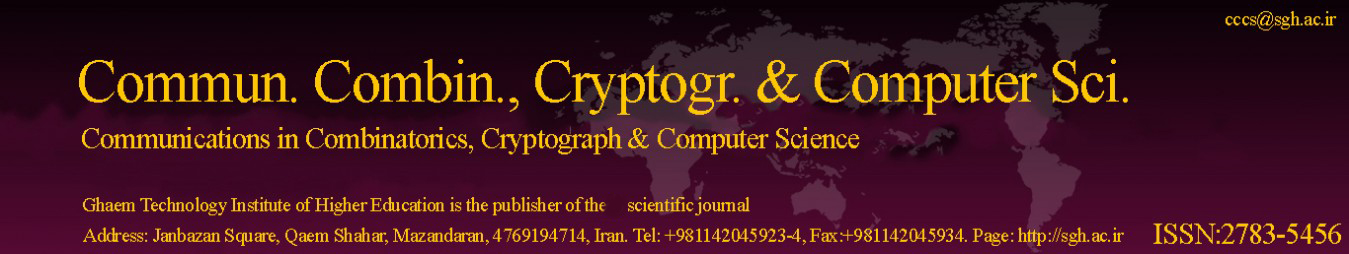Original Research Article

Article volume = 2021 and issue = 1

Pages: 92–96

Article publication Date: November, 1, 2021

# Laplacian Energy of The Conjugacy Class Graphs of Metabelian Groups of Order Less Than 3

#### Zeinab Foruzanfar, Mehdi Rezaei

Imam Khomeini International University - Buin Zahra Higher Education Center of Engineering and Technology, Qazvin, Iran.

##### Abstract:

Let G be a finite group and V(G) be the set of all non-central conjugacy classes of G. The conjugacy class graph 􀀀 (G) is defined as: its vertex set is the set V(G) and two distinct vertices xG and yG are connected with an edge if (o(x), o(y)) > 1. In this paper, we compute the Laplacian energy of the conjugacy class graphs of metabelian groups of order less than thirty

##### Keywords:

Metabelian group, conjugacy class graph, Laplacian energy, eigenvalue.

##### References:
•  R. B. Bapat, Graphs and Matrices, Springer, New York, (2010). 2.3
•  L. W. Beineke, R. J. Wilson, Topics in algebraic graph theory, 102, Cambridge University Press, New York, (2004). 2.2
•  W. B. Fite, On metabelian groups, Trans. Amer. Math. Soc. 3 (1902), 331–353. 1
•  X. You, G. Qian, A new graph related to conjugacy classes of finite groups, (Chinese) Chinese Ann. Math. Ser. A, 28 (2007), 631–636. 1